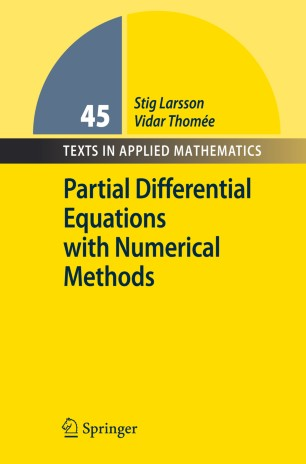Partial Differential Equations with Numerical Methods

• Vidar ThoméeTextbook

Part of the Texts in Applied Mathematics book series (TAM, volume 45)

1. Front Matter
Pages I-XI
2. Pages 1-14
3. Pages 15-24
4. Pages 25-41
5. Pages 77-94
6. Pages 109-127
7. Pages 129-148
8. Pages 149-161
9. Pages 163-183
10. Pages 185-199
11. Pages 201-216
12. Pages 217-224
13. Back Matter
Pages 225-261

Introduction

The book is suitable for advanced undergraduate and beginning graduate students of applied mathematics and engineering. The main theme is the integration of the theory of linear PDEs and the numerical solution of such equations. For each type of PDE, elliptic, parabolic, and hyperbolic, the text contains one chapter on the mathematical theory of the differential equation, followed by one chapter on finite difference methods and one on finite element methods. As preparation, the two-point boundary value problem and the initial-value problem for ODEs are discussed in separate chapters. There is also one chapter on the elliptic eigenvalue problem and eigenfunction expansion. The presentation does not presume a deep knowledge of mathematical and functional analysis. Some background on linear functional analysis and Sobolev spaces, and also on numerical linear algebra, is reviewed in two appendices.

Keywords

Boundary value problem Sobolev space elliptic equation finite difference method finite element method hyperbolic equation parabolic equation partial differential equation

Authors and affiliations

• 1
• Vidar Thomée
• 1
1. 1.Department of Mathematics SciencesChalmers University of Technology and University of GothenburgGöteborgSweden

Bibliographic information

• DOI https://doi.org/10.1007/978-3-540-88706-5
• Copyright Information Springer Berlin Heidelberg 2003
• Publisher Name Springer, Berlin, Heidelberg
• eBook Packages
• Print ISBN 978-3-540-88705-8
• Online ISBN 978-3-540-88706-5
• Series Print ISSN 0939-2475
• Buy this book on publisher's site